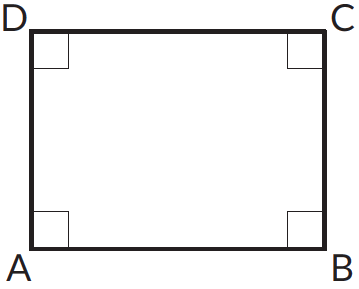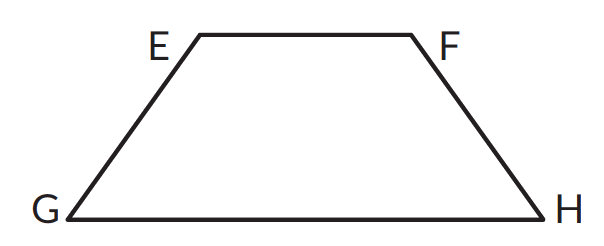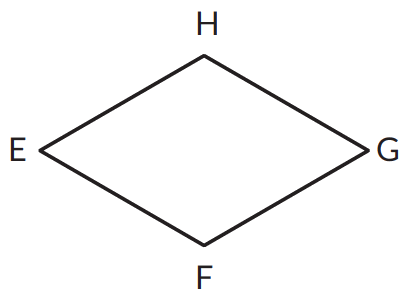Go back to  'Geometry'

A quadrilateral is a polygon with 4 sides and 4 vertices.

SquareProperties of a square

A square has

• four equal sides - $$\text {Side } AB = \text {Side } BC = \text {Side } CD = \text {Side } AD$$
• four right angles - \begin{align} \angle A = \angle B = \angle C = \angle D = \,90^\circ \end{align}
• two pairs of parallel sides - \begin{align} AB ∥ DC \qquad AD ∥ BC \end{align}

RectangleProperties of a rectangle

A rectangle has

• two pairs of parallel sides - \begin{align} AB ∥ DC \qquad AD ∥ BC \end{align}
• four right angles - \begin{align} \angle A = \angle B = \angle C = \angle D = \,90^\circ \end{align}
• opposite sides of equal length - $$AD = BC$$ & $$AB = DC$$

ParallelogramProperties of a parallelogram

• two pairs of parallel sides \begin{align}PQ ∥ RT \qquad PR ∥ QT \end{align}
• opposite sides are of equal length. $$\text{Side }PQ = \text{Side }RT$$  and  $$\text{Side }PR = \text{Side }QT$$

TrapeziumProperties of a trapezium

• One pair of parallel sides. \begin{align} EH ∥ GH \end{align}

RhombusA rhombus has

• two pairs of parallel sides.  \begin{align} EH ∥ FG \qquad \qquad EF ∥ HG \end{align}
• four equal sides. $$\text{Side }EH = \text{Side }HG = \text{Side }GF = \text{Side }FE$$
• Opposite angles are equal. \begin{align} \angle H = \angle F = \angle E = \angle G \end{align}

## Angle sum property of quadrilaterals

The sum of the angles of a quadrilateral is always $$360^\circ$$. This property is referred to as the angle sum property of a quadrilateral.In the figure given above the sum of angles $$A$$, $$B$$, $$C$$ & $$D$$ is $$360^\circ$$. This property comes handy when calculating unknown measure of angles in a quadrilateral.

## Tips and Tricks

• Tip: While naming a quadrilateral you can name it in anti-clockwise direction. For example, in the figure given below the quadrilateral can be named as $$\square \,ABCD$$ and $$\square \,ACBD$$.• While calculating the measures of an unknown angles in a quadrilateral dividing the quadrilateral into two triangles would help.

### Common mistakes or misconceptions

Misconception 1: Any four sided figure is a quadrilateral. For example, the figure given below is a quadrilateral.Though the above given has 4 sides and is a closed figure, it is not a quadrilateral. Quadrilaterals are closed figures made of non-intersecting line segments.

Misconception 2: All rectangles are squares
Many rectangles do not have 4 equal sides and 4 equal angles. However, all squares are rectangles as they all have 4 right angles, their opposite sides are parallel to each other and opposite sides are equal to each other.

Misconception 3: All rhombuses are squares
Many rhombuses do not have four equal sides and four equal angles. However, all squares are rhombuses as it has 4 equal sides, its opposite sides are parallel to each other.

1.This is ______________________

1.This is ______________________

1.This is ______________________

1.This is ______________________

1.This is ______________________